# Square Root Function

## LabVIEW 2018 Help

Edition Date: March 2018
Part Number: 371361R-01
View Product InfoLabVIEW 2015 HelpLabVIEW 2016 HelpLabVIEW 2017 HelpLabVIEW 2018 HelpLabVIEW 2019 Help

Owning Palette: Numeric Functions

Requires: Base Development System

Computes the square root of the input value.

If x is negative, the square root is NaN unless x is complex. If x is a matrix, this function takes the matrix square root of x. The connector pane displays the default data types for this polymorphic function.Note  If you wire a value that has a unit with an odd exponent to the square root function, the wire breaks because LabVIEW does not support units with fractional exponents. For example, 15m2 is an acceptable input value, but 15m3 is not an acceptable input value.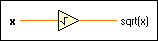x can be a scalar number, array or cluster of numbers, array of clusters of numbers, and so on.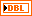sqrt(x) is a double-precision, floating-point number if x is an integer. If x is less than 0, sqrt(x) is not a number (NaN), unless x is complex. When x is of the form x = a + bi, that is, when x is complex, the function defines the magnitude and phase with the following equations: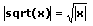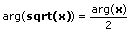where |x| is the magnitude of x and arg(x) is the phase of x: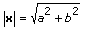arg(x) = arctan2(b,a)Note  You can manually configure this function to output data of a type you want. To specify the output data type, right-click the function and select Properties to display the Object Properties dialog box. On the Output Configuration page, click the Representation icon and select the data type you want. A blue coercion dot appears on the output terminal of the function to indicate that you have configured the output data type.

## Square Root Details

When you wire matrix data as an input to this function, a VI that includes subVIs that work with the matrix data type replaces the function. The resulting VI has the same icon but contains a matrix-specific algorithm. The node remains a VI if you disconnect the matrix from the input(s). Wire other data types as inputs to restore the original function. If you wire a data type to a function and that data type causes a basic math operation to fail, the function returns an empty matrix or NaN.